# C Program to implement Radix sort Algorithm

In this tutorial you will learn about the C Program to implement Radix sort Algorithm and its application with practical example.

## C Program to implement Radix sort Algorithm

In this tutorial, we will learn to create a C program that will do Radix sort using C programming.

## Prerequisites

Before starting with this tutorial we assume that you are best aware of the following C programming topics:

• Operators in C Programming.
• Basic Input and Output function in C Programming.
• Basic C programming.
• For loop in C Programming.
• Creating and Using the user-defined function in C programming.

In every programming language, the sorting of data is a very important factor. Sorting works are done mainly with the techniques available in C Language. Many different techniques for sorting are available to work with but in this tutorial, we will focus on the Radix Sort Technique.

The Radix Sort is a simple and easy technique. It first makes the group of similar values from a big given array then those small groups are again re-arranged and placed at the deserving positions.

Output:-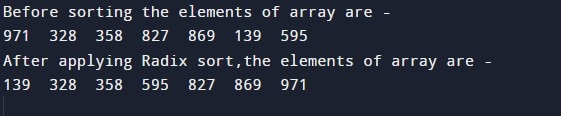In the above program we have first initialized the required variable and passed them to other functions for the Radix sort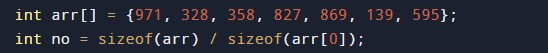• no = it will hold the size of the array.
• arr[]= it will hold the elements in an array.
• i= it will hold the integer value to control the array.
• j= it will hold the integer value to control the array.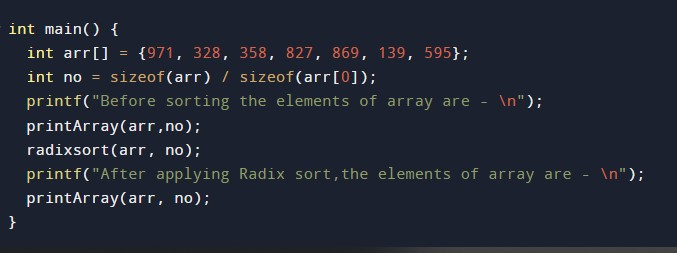Now the program will call the print function to display the elements.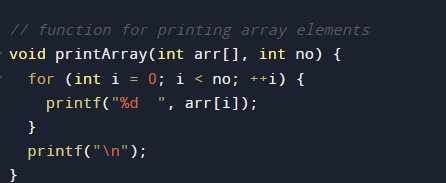After this, the program executes the next function & finds the largest number from the elements of the array.Now the function to do count sort first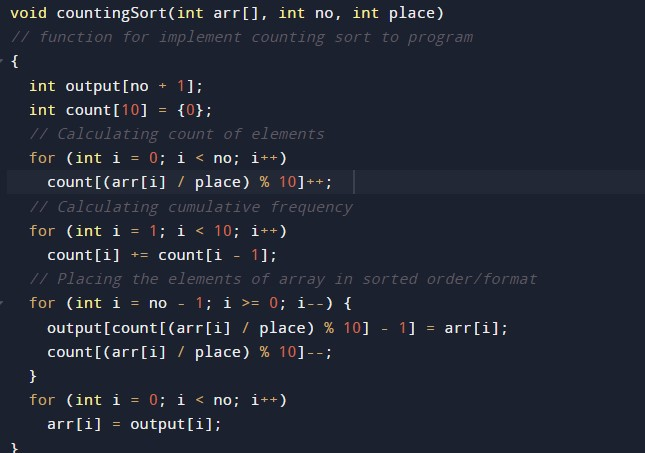Now, the final step radix function to process the data.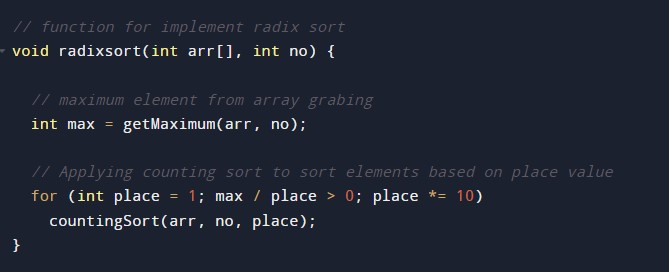As a result we got the sorted array.

By calling the print function.

In this tutorial we have learn about the C Program to implement Radix sort Algorithm and its application with practical example. I hope you will like this tutorial.